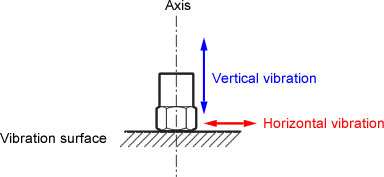Accelerometer & related products FAQWhat is the lateral direction sensitivity of single axis accelerometer?An expression such as "Lateral-direction sensitivity: 5% or less" is provided in the instruction manuals. To measure vertical vibration, the accelerometer is fixed vertically against the vibration surface as shown below. If the vibration of the horizontal direction (lateral-direction) is large, it impacts the voltage output of accelerometer. In instruction manuals, the degree of impact is represented as lateral-direction sensitivity and is described such as "5% or less of maximum vertical sensitivity". In the case of accelerometer that is indicated as "5% or less of maximum vertical sensitivity", where there is 1m/s2 of acceleration in the horizontal direction in addition to the vertical vibration, the maximum vertical sensitivity of 5%, which is the voltage equivalent to 0.05m/s2, is included in the output as the error. When the vibration in the horizontal direction is also large, for measurement, it is recommended to use 3-axis accelerometer such as NP-3560B that is capable of measuring acceleration in the horizontal direction also.Revised:2007/02/19